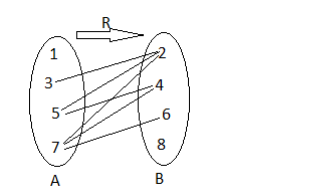# Let A = {1, 3, 5, 7} and B = {2, 4, 6, 8}.

Question:

Let $A=\{1,3,5,7\}$ and $B=\{2,4,6,8\}$

Let $R=\{(x, y),: x \in A, y \in B$ and $x>y\} .$

(i) Write $\mathbf{R}$ in roster form.

(ii) Find dom (R) and range (R).

(iii) Depict $\mathbf{R}$ by an arrow diagram.

Solution:

Given: A = {1, 3, 5, 7} and B = {2, 4, 6, 8}

(i) $R=\{(x, y),: x \in A, y \in B$ and $x>y\}$

So, R in Roster Form,

R = {(3, 2), (5, 2), (5, 4), (7, 2), (7, 4), (7, 6)}

(ii) $\operatorname{Dom}(\mathrm{R})=\{3,5,7\}$

Range(R) = {2, 4, 6}

(iii)